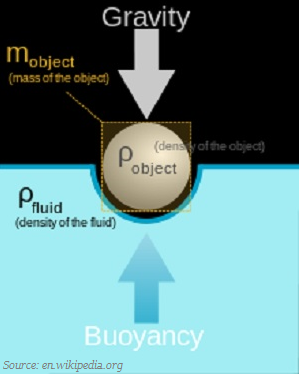# Physics - Mass and Weight

## Introduction

• The mass of an object is always constant and does not change from place to place.• The mass remains same whether the object is on the earth, the moon, or even in outer space.

• The force of attraction of the earth (due to gravitational force) on an object is called as the weight of the object.

• Weight is denoted by English capital letter ‘W.’

• Weight is calculated as −

$$W = m \: \times \: g$$

Where,

• m is equal to mass of the object.

• g is equal to the acceleration due to gravity.

• The SI unit of weight is the same as that of force, i.e., newton (N).

• Since, weight of an object is dependent on certain factors; therefore, weight is changeable (depending upon the place where it is), but mass of the same object remains constant irrespective of its location (where it is).

## Thrust

• The force that acts on an object perpendicular to the surface is known as thrust.• The effects of forces of the same magnitude on different areas are different because the effect of thrust depends on the area on which it acts.

• For example, if someone is standing on loose sandy surface, then the force, that is, the weight of his body is acting on an area equal to area of his feet. But when he lies down on the same surface, the same force acts on an area equal to the contact area of his whole body, which is larger than the area of his feet. Thus the effect of thrust on sandy surface is larger while standing than while lying.

## Pressure

• The thrust on unit area is known as pressure. It is calculated as −

$$Pressure = \frac{Thrust}{Area}$$

• The SI unit of pressure is known as pascal, symbolized as Pa.

• The same force, if acting on a smaller area, exerts a larger pressure, but if acting on a larger area, exerts smaller pressure. Because of this reason - a nail has a pointed tip, knives have sharp edges, etc.

## Buoyancy

• The upward force exerted by the water molecules on the object is known as up thrust or buoyant force.• The magnitude of the buoyant force depends on the density of the water/fluid.

• The objects, which have the density lesser than that of water/liquid float on the water/liquid; whereas, the objects, which have the density greater than that of water/liquid sink in the water/liquid.

• Mass of a unit volume is known as density.

## Archimedes’ Principle

• The force of buoyancy was first time noticed by Archimedes (a Greek Scientist) and to explain the phenomenon, he proposed a principle known as Archimedes’ Principle.• Archimedes’ principle“When a body is immersed fully or partially in a fluid, it experiences an upward force that is equal to the weight of the fluid displaced by it.”